# Ossona de Mendez, Patrice

## Linear Hypergraphs with Dimension 3 ★★

Author(s): de Fraysseix; Ossona de Mendez; Rosenstiehl

Conjecture   Any linear hypergraph with incidence poset of dimension at most 3 is the intersection hypergraph of a family of triangles and segments in the plane.

Keywords: Hypergraphs

## Straight line representation of planar linear hypergraphs ★★

Author(s): de Fraysseix; Ossona de Mendez

Conjecture   Every planar linear hypergraph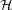has a straight line representation in the plane which maps each vertexto a point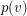and each edge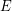to a straight line segment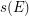, in such a way that:
\item for each vertexand each edge, we have:\item for each couple of distinct edges, we have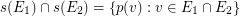Keywords: intersection graph; planar hypergraph